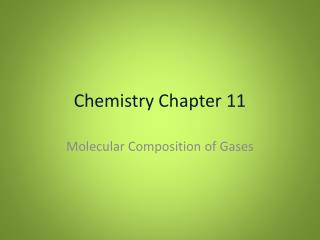DownloadDownload PresentationChemistry Chapter 11

# Chemistry Chapter 11

Télécharger la présentation## Chemistry Chapter 11

- - - - - - - - - - - - - - - - - - - - - - - - - - - E N D - - - - - - - - - - - - - - - - - - - - - - - - - - -
##### Presentation Transcript

1. Chemistry Chapter 11 Molecular Composition of Gases

2. Volume and mass • Gay-Lussac examined gas volume in reactions • Noted: 2 L H2 and 1 L O2 can form 2 L water vapor • 2:1:2 volume relationship of H:O: water • Simple definite proportions hold true for other gases in reactions • this lead to ….

3. Gay-Lussac’s Law of Combining Gas Volumes • At constant temperature and pressure the volumes of gaseous reactants and products can be expressed as ratios of small whole numbers

4. Avogadro (again) • Combining volumes seemed to challenge the indivisibility of the atom… • Avogadro posited that some molecules might contain more than one atom (ex. O2 explains the 2:1:2 H:O:water ratio) • Avogadro's Law: Equal volumes of gases at the same temperature and pressure contain equal numbers of molecules

5. Implications of Avogadro • At the same temperature and pressure the volume of a gas varies directly with the number of molecules • Avogadro believed that some elements must exist in diatomic form (H2, O2, N2)

6. Avogadro continued • H, O and water illustrate this well • 2 volumes H2 + 1 volume O2 = 2 volumes H2O Leads to the balanced equation… 2H2 + O2 2H2O • Which confirms the diatomic molecule hunch nicely!

7. Avogadro in algebra • Gas volume is directly proportional to the amount of gas (number of particles) at a given temperature and pressure give us: V = kn • Where • V is volume • n is the amount of gas (in moles) • k is a constant

8. Molar Volumes • One mole of gas at STP will occupy 22.4 L • 1 mole/ 22.4 L of ________gas can be used as a conversion factor to find number of particles, mass, or volume of a gas at STP Practice! • Problems 1-3 page 337

9. 11-2 Ideal Gas Law • A mathematical relationship among pressure, volume, temperature and number of moles • To derive (see p. 341) • Ideal gas law: • V= nRT/P OR PV = nRT • V is volume, P is pressure, T is temperature, n is number of moles, and R is a constant

10. The Ideal Gas Constant • R= (1 atm) (22.4 L)/(1 mol) (273.15 K) Or • R= 0.08205784 L x atm / mol x K • (round to .0821) • USE ONLY when units are appropriate! • For any other units see chart on p. 342 • Practice Problems! p. 345

11. Finding Molar Mass or Density • Use V= nRT/P but remember that n (number of moles) is equal to mass (m) /molar mass (M) Substituting gives: PV= mRT/M or M= mRT/PV • Density is just mass (m) per unit volume (V) Substituting gives: M= DRT/P or D= MP/RT Practice problems! 1-4 page 346

12. 11-3 Stoichiometry of gases • Volume ratios of gases in reactions can be used exactly as mole ratios are in standard mass-mole, mass-mass, mole mole etc. problems • Practice! 1-2 pg. 348

13. More Stoich! • When given a volume for a reactant and a mass for a reactant… • Go through moles • Need conditions (temp, pressure, etc) for each gas • Ideal gas law works well for this • Practice problems! #1-2, p.349 # 1-2 p.350

14. Effusion and Diffusion • Rates of either can be calculated! • Remember! KE= ½ m v2 • And… for any two gases (A & B, lets say) at the same temp KEA = KEB so… ½ MA vA2 = ½ MBvB2 • Where M is molar mass and v is molecular velocity • You can multiply by 2 to clean up and get MA vA2 = MBvB2

15. Effusion and diffusion (con’t) • Recall: MA vA2 = MB vB2 • Rearrange: vA2 / vB2 = MB / MA • Take square roots: vA / vB = √MB / √MA • Because rate of effusion is directly proportional to molecular velocity we can say that: rate of effusionA / rate of effusionB = √MB / √MA

16. Grahams Law of effusion • Rates of effusion of gases at the same temperature and pressure are inversely proportional to the square roots of their molar masses • So? Density varies directly with molar mass so… rate of effusionA / rate of effusionB = √DB / √DA (Where D is density)

17. Grahams… • Can be used to find density or molar mass of gases effusing. • Practice problems! #1-3 p.355

18. That’s all folks!